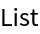Function Repository Resource:

# PolynomialSystemInfeasibilityCertificate

Find an independently verifiable certificate proving that a polynomial system has no solutions

 ResourceFunction["PolynomialSystemInfeasibilityCertificate"][{f1,…,fk},{g1,…,gm},vars,Reals] finds an infeasibility certificate for the real system f1==0∧…∧fk==0∧g1>=0∧…∧gm>=0. ResourceFunction["PolynomialSystemInfeasibilityCertificate"][{f1,…,fk},{g1,…,gm},vars,Complexes] finds an infeasibility certificate for the complex system f1==0∧…∧fk==0∧g1!=0∧…∧gm!=0.

## Details

ResourceFunction["PolynomialSystemInfeasibilityCertificate"] is typically used to find an independently verifiable certificate proving that a system of real polynomial equations and inequalities does not have real solutions or a system of complex polynomial equations and inequations does not have complex solutions.
ResourceFunction["PolynomialSystemInfeasibilityCertificate"][{f1,,fk},{g1,,gm},vars,Reals] requires {f1,,fk} and {g1,,gm} to be polynomials in vars with real numeric coefficients.
An infeasibility certificate for a real system has the form {{t1,,tr},{u1,,uk}}. Each ti is a triple {qi,μi,αi}, where qi is a real symmetric positive semidefinite matrix, μi is a list of monomials in vars, and αi is a subset of {1,,m}. The {u1,,uk} are polynomials in vars with real numeric coefficients. Let si=μi.qi.μi and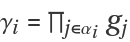. Then si is non-negative for all values vars. The certificate satisfies the condition 1+i=1rsiγi==u1f1++ukfk. Note that at any real solution of the system f1==0∧fk==0∧g1>=0∧gm>=0 we would have 1+i=1rsiγi>=1 and u1f1++ukfk==0, and hence the system has no real solutions.
ResourceFunction["PolynomialSystemInfeasibilityCertificate"][{f1,,fk},{g1,,gm},vars,Complexes] requires {f1,,fk} and {g1,,gm} to be polynomials in vars with numeric coefficients.
An infeasibility certificate for a complex system has the form {{u1,,uk},{e1,,em}}, where {u1,,uk} are polynomials in vars with numeric coefficients and {e1,,em} are non-negative integers. The certificate satisfies the condition u1f1++ukfk==g1e1··gmem. Note that at any solution of the system f1==0∧fk==0∧g1!=0∧gm!=0 we would have u1f1++ukfk==0 and g1e1··gmem!=0, and hence the system has no solutions.

## Examples

### Basic Examples (2)

Find an infeasibility certificate for the real system x2+y2=1 ∧ x4+y4≤1/4:

 In:=Out=Verify that the matrices qi are positive semidefinite:

 In:=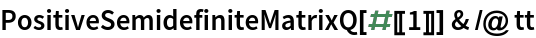Out=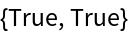Verify the condition 1+∑i=1rsiγi==u1f1++ukfk:

 In:=Out=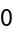Find an infeasibility certificate for the complex system x4+y4+z4=1 ∧ 2 x2 y2+z4=1 ∧ x≠y ∧ x≠-y:

 In:=Out=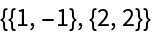Verify the condition u1f1++ukfk==g1e1··gmem:

 In:=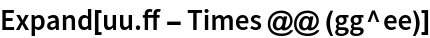Out=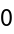We obtain a similar result by creating a new equation that forces both inequations to be False and removing the inequations:

 In:=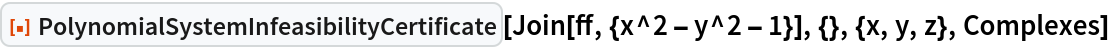Out=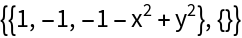### Scope (6)

Infeasibility certificate for a real system of equations:

 In:=Verify correctness of the certificate:

 In:=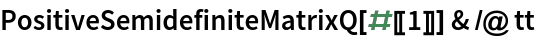Out=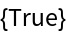In:=Out=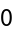Visualize the disjoint solution surfaces of the equations:

 In:=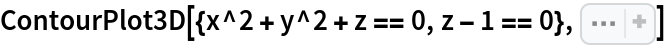Out=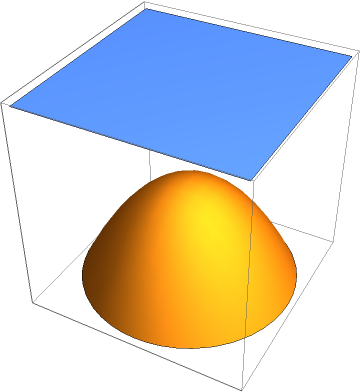Infeasibility certificate for a real system of inequalities:

 In:=Verify correctness of the certificate:

 In:=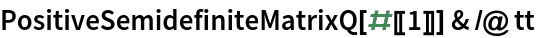Out=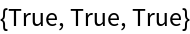In:=Out=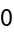Visualize the disjoint solution regions of the inequalities:

 In:=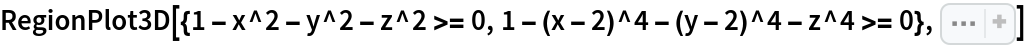Out=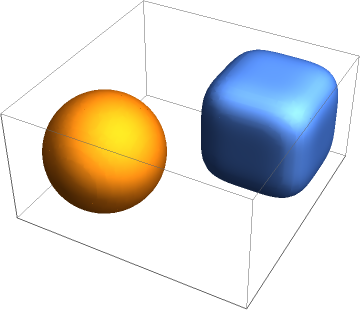Infeasibility certificate for a real system of equations and inequalities:

 In:=Verify correctness of the certificate:

 In:=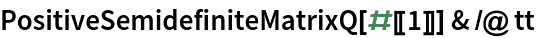Out=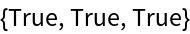In:=Out=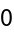Visualize the disjoint solution sets of the equation and of the inequalities:

 In:=Out=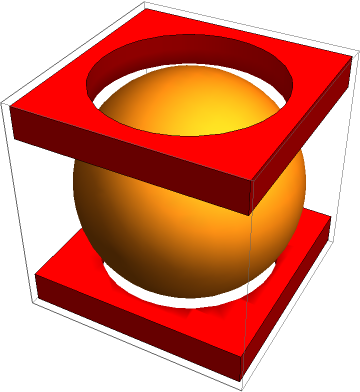Find an infeasibility certificate for the complex system of equations:

 In:=Out=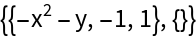Verify correctness of the certificate:

 In:=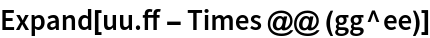Out=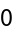Find an infeasibility certificate for the complex system of equations and inequations:

 In:=Out=Verify correctness of the certificate:

 In:=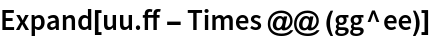Out=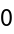PolynomialSystemInfeasibilityCertificate returns unevaluated when it fails to find an infeasibility certificate:

 In:=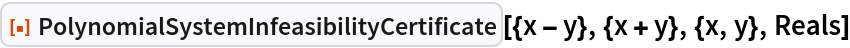Out=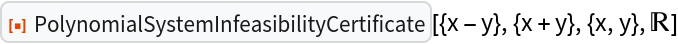In this case the system has solutions:

 In:=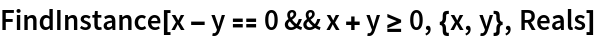Out=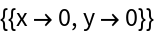### Applications (3)

Find an independently verifiable proof that surfaces x2+y2+z2=1 and x4+y4+z4=1/4 are disjoint:

 In:=Verify the proof:

 In:=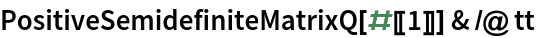Out=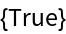In:=Out=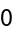Visualize the surfaces:

 In:=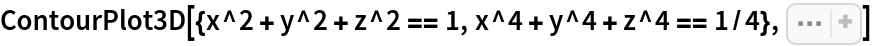Out=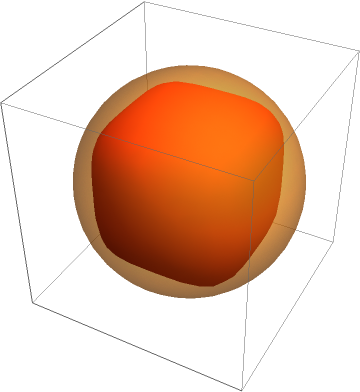Find a proof that solids z2≥2 x2+2 y2 and 16 (x2+y2)≥(x2+y2+z2+2)2 are disjoint:

 In:=Verify the proof:

 In:=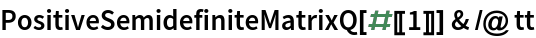Out=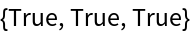In:=Out=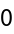Visualize the solids:

 In:=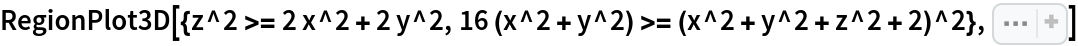Out=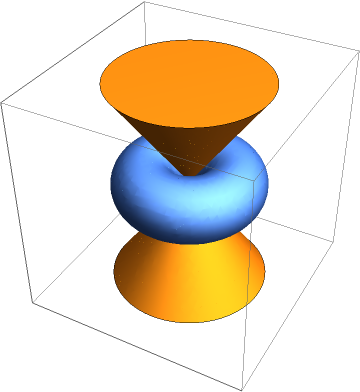Find a proof that x+y+z belongs to the radical of ⟨x3+y3+z3,(x+y)2 (x+z)2 (y+z)2:

 In:=Out=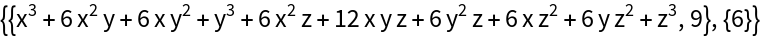Verify that (x+y+z)e==u(x3+y3+z3)+v((x+y)2 (x+z)2 (y+z)2):

 In:=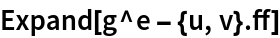Out=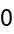### Properties and Relations (4)

PolynomialSystemInfeasibilityCertificate finds an independently verifiable certificate of infeasibility:

 In:=Out=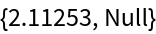Verify correctness of the certificate:

 In:=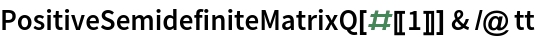Out=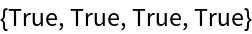In:=Out=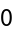FindInstance usually faster proves infeasibility, but does not provide a certificate:

 In:=Out=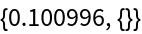You can also prove infeasibility using Resolve:

 In:=Out=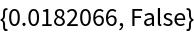### Possible Issues (3)

PolynomialSystemInfeasibilityCertificate may fail even if the problem is infeasible:

 In:=Out=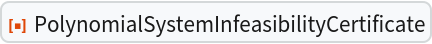This shows that x2+y2-1=0∧-x3+2 y3-2-ε>=0 is infeasible for any ε>0:

 In:=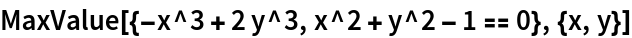Out=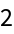For a larger value of ε PolynomialSystemInfeasibilityCertificate succeeds:

 In:=Out=